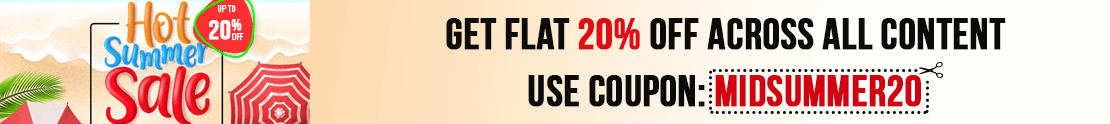# User ForumSubject :IMO    Class : Class 4

## Ans 1:

Class : Class 7
The answer may be park as - You're asked Akshat is facing the mall Now he took a 3/4 turn anti-clockwise, so he is facing the Police Post Now he takes a 1/2 turn clockwise, so now he is facing the park Therefore the answer is 'A Park'

Class : Class 4

## Ans 3:

Class : Class 6

Subject :IMO    Class : Class 5

## Ans 1:

Class : Class 5
I think the answer is D

Subject :IMO    Class : Class 8

## Ans 1:

Class : Class 9
square all terms= x2 + 1/x2 +2=16 x2+1/x2=14 x-1/x=? square all = x2+1/x2 - 2 = ? x2-1/x2=14 thus- 14 -2 = 12 root of 12 is 2 root 3.

## Ans 2:

Class : Class 8
(A)

Subject :IMO    Class : Class 9

## Ans 1:

Class : Class 9
((a+b)^2-(a-b)^2)/ab=4

Subject :IMO    Class : Class 7

Class : Class 1

Subject :IMO    Class : Class 7

## Ans 1:

Class :
FIRST MAKE THAT SIMILAR 4 TRAINGLES INSIDE A RECTANGLE WHOSE TOTAL AREA IS 28 CM SQUARE NOW WE ARE LEFT WITH FOUR EQUALITERAL TRAINGLES ARE OF WHOSE ONE IS 1/2*L/2*B/2=LB/8 FOR FOUR TRAINGLES TOTAL AREA =4*LB/8=LB/2 NOW LB=LB/2+28 LB/2=28 LB=56 CM SQAURE

## Ans 2:

Class : Class 9
how is the answer worked out?

Subject :IMO    Class : Class 6

Class : Class 6
plz explain

Class : Class 6

Class : Class 6
C.) 75

## Ans 4:

Class : Class 6

Subject :IMO    Class : Class 3

Class : Class 8

Class : Class 6

## Ans 3:

Class : Class 6
Correct ans is c. But it is shown as wrong . Please correct

## Ans 4:

Class : Class 8
c

Subject :IMO    Class : Class 3

## Ans 1:

Class : Class 2
Yes, 12-11=1The answer given is wrong

Class : Class 6

## Ans 3:

Class : Class 6
yes it is 12 - 11 solution is wrong

Class : Class 3

## Ans 5:

Class : Class 4
ANS. WRONG STRAIGHT LINES-12 TRIANGLES-11 12-11=1

## Ans 6:

Class : Class 4
yes 12-11 = 1 is the solution

Class : Class 1

## Ans 8:

Class : Class 4
solution is wrong

Class : Class 4

Class : Class 4

## Ans 11:

Class : Class 3
the answer is incorrect. It should be 12-11 = 1

## Ans 12:

Class : Class 4
12-11=1, so the answer should be D.

Class : Class 5

Class : Class 5

Class : Class 2

Class : Class 5

## Ans 17:

Class : Class 4
Yes number of straight line is 12 and number of triangle is 11 so 12-11=1

## Ans 18:

Class : Class 2
Solution is wrong

Class : Class 4
Ans. is 12-11=1

Class : Class 5

## Ans 21:

Class : Class 8
yes, it should be 12-11 = 1

Subject :IMO    Class : Class 4

## Ans 1:

Class : Class 10
Number of Rs. 10 Notes only: 260/10 = 26 Number of Rs. 5 Notes only: 260/10 = 52 Therefore Rs. 5 notes more than Rs. 10, 52-26=26 Option (B)

## Ans 2:

Class : Class 6
AFTER THE SOLUTION I FOUND IT VERY EASY

Class : Class 6

Class : Class 5